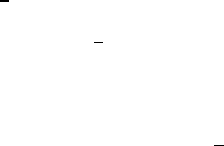Class Notes (1,100,000)
CA (630,000)
UTSG (50,000)
MAT (4,000)
MAT136H1 (900)
all (200)
Lecture

# 5.4 Integration & Anti-derivatives Indefinite Integrals Question #1 (Easy)

Department
Mathematics
Course Code
MAT136H1
Professor
all

Page:
of 15.4 Integration & Anti-derivatives
Indefinite Integrals
Question #1 (Easy): Finding the General Indefinite Integral
Strategy
The notation for the general indefinite integral is:    . This represents the entire
anti-derivative family of .
So, always remember to add the constant term after the anti-derivative function .
Sample Question
Find the general indefinite integral.
  
Solution
1) This integral can be split into two integrals based on one of the properties of integrals:
So then,     
2) Take the anti-derivate of each component:
    and 
 
3) Putting it together:     
 
Though two constant factors  are added, they represent an arbitrary number and so it can be
simply written as another .
Therefore, the general indefinite integral of     
 .